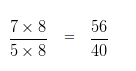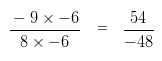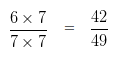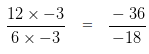# Equivalent Rational Numbers

1. To obtain a equivalent rational number of the given rational number we have to multiply the numerator and denominator of a given rational number by the same nonzero number.

2. To obtain a rational number equivalent to the given rational number we have to divide the numerator and denominator of a given rational number by a common divisor.

### Equivalent Rational Numbers Examples

Example 1

Are 6/8 and 9/12 equivalent Rational Numbers?

Explanation

If two Rational Numbers are equivalent, the product obtained by Cross Multiplying them would also be equal

On Cross Multiplying the given Rational numbers

6/8 and 9/12

we get,

6 x 12 = 72

and 9 x 8 = 72

Since, 6 x 12 = 9 x 8

So, 6/8 = 9/12

Hence, 6/8 and 9/12 are equivalent Rational Number.

Example 2

Are 14/21 and 12/15 are equivalent Rational Numbers?

Explanation

If two Rational Numbers are equivalent, the product obtained by Cross Multiplying them would also be equal

On Cross Multiplying the given Rational numbers

14/21 and 12/15

we get,

14 x 15 = 210

and 12 x 21 = 252

Since, 14 x 15 ≠ 12 x 21

So, 14/21 ≠ 12/15

Hence, 14/21 and 12/15 are not equivalent Rational Number.

### Equivalent Rational Number with given Numerator Examples

Example 3

Express 7/5 as a Rational Number with Numerator 56.

Explanation

Numerator of 7/5 = 7

We need to change the Numerator of 7/5 to 56

We need to find a number, with which we should multiply 7 so it is equal to 56

To obtain that number, we would need to divide 56 by 7 i.e,

56/7 = 8

So, we have to multiply both the Numerator and Denominator of given Rational Number by 8Hence, 7/5 can be expressed as 56/40

Example 4

Express (-9)/8 as a Rational Number with Numerator 54.

Explanation

Numerator of (-9)/8 = -9

We need to change the Numerator of (-9)/8 to 54

We need to find a number, with which we should multiply -9 so it is equal to 54

To obtain that number, we would need to divide 54 by -9 i.e,

54/(-9) = -6

So, we have to multiply both the Numerator and Denominator of given Rational Number by -6Hence, (-9)/8 can be expressed as 54/(-48)

### Equivalent Rational Number with given Denominator Examples

Example 5

Express 6/7 as a Rational Number with Denominator 49.

Explanation

Denominator of 6/7 = 7

We need to change the Denominator of 6/7 to 49

We need to find a number, with which we should multiply 7 so it is equal to 49

To obtain that number, we would need to divide 49 by 7 i.e,

49/7 = 7

So, we have to multiply both the Numerator and Denominator of given Rational Number by 7Hence, 6/7 can be expressed as 42/49

Example 6

Express 12/6 as a Rational Number with Denominator -18.

Explanation

Denominator of 12/6 = 6

We need to change the Denominator of 12/6 to -18

We need to find a number, with which we should multiply 6 so it is equal to -18

To obtain that number, we would need to divide -18 by 6 i.e,

(-18)/6 = -3

So, we have to multiply both the Numerator and Denominator of given Rational Number by -3Hence, 12/6 can be expressed as (-36)/(-18)